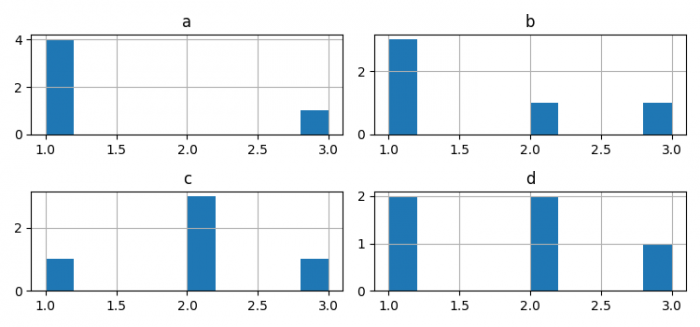# How to plot histograms from dataframes in Pandas using Matplotlib?

To plot histograms against Pandas/Matplotlib, we can take the following steps −

• Set the figure size and adjust the padding between and around the subplots.
• Make a potentially hetrogeneous tabular data using Pandas dataframe.
• Use the dataframe to make a histogram.
• To display the figure, use show() method.

## Example

from matplotlib import pyplot as plt
import pandas as pd

plt.rcParams["figure.figsize"] = [7.50, 3.50]
plt.rcParams["figure.autolayout"] = True

df = pd.DataFrame({'a': [1, 1, 1, 1, 3],
'b': [1, 1, 2, 1, 3],
'c': [2, 2, 2, 1, 3],
'd': [2, 1, 2, 1, 3],
})
df.hist()

plt.show()

## Output